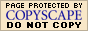"Number" Word Problems"Number" word problems are fairly contrived, but they're also fairly standard, so you should learn how to handle them. After all, the point of these problems isn't their relation to "real life", but your ability to extract the math from the English.

• The sum of two consecutive integers is 15. Find the numbers.

They've given me two pieces of information here. First, I know that I am adding two numbers, and their sum is fifteen. Second, I know that the numbers are nice neat round numbers (like –3 or 6), not messy ones (like –4.628 or 17/32), and that the second number is one more than the first. This last piece of information comes from the fact that "consecutive integers" (or "consecutive whole numbers", if they're restricting the possibilities to only positive numbers) are one unit apart. Examples of "consecutive integers" would be –12 and –11, 1 and 2, and 99 and 100. Using these facts, I can set up the translation.

I will represent the first number by "n". Then the second number has to be "n + 1". Their sum is then:

n + (n + 1) = 15
2n + 1 = 15

2n = 14

n = 7

The exercise did not ask me for the value of the variable n; it asked for the identity of two numbers. So my answer is not "n = 7"; the actual answer is:

"The numbers are 7 and 8."

• The product of two consecutive negative even integers is 24. Find the numbers.

They have told me quite a bit about these two numbers: the numbers are even and they are negative. (The fact that they are negative may help if I come up with two solutions — a positive and a negative — so I'll know which one to pick.) Since even numbers are two apart (for example, –4 and –2 or 10 and 12), then I also know that the second number is two greater than the first. I also know that, when I multiply the two numbers, I will get 24. In other words, letting the first number be "n" and the second number be "n + 2", I have:

(n)(n + 2) = 24
n2 + 2n = 24

n2 + 2n – 24 = 0

(n + 6)(n – 4) = 0

Then the solutions are n = –6 and n = 4. Since the numbers I am looking for are negative, I can ignore the "4" and take n = –6. Then the next number is n + 2 = –4, and the answer is

The numbers are –6 and –4.

In the exercise above, one of the answers was one of the solutions to the equation; the other answer was the negative of the other solution to the equation. Warning: Do not assume that you can use both solutions if you just change the signs to be whatever you feel like. While this often "works", it does not always work, and it's sure to annoy your teacher. Throw out invalid results, and solve properly for valid ones.

• Twice the larger of two numbers is three more than five times the smaller, and the sum of four times the larger and three times the smaller is 71. What are the numbers?

The point of exercises like this is to give you practice in unwrapping and unwinding these words, and turning the words into algebraic equations. The point is in the solving, not in the relative "reality" of the problem. That said, how do you solve this? The best first step is to start labelling:

the larger number:  x
the smaller number:
y

twice the larger:  2x
three more than five times the smaller:
5y + 3
relationship between ("is"):
2x = 5y + 3

four times the larger:  4x
three times the smaller:
3y
relationship between ("sum of"):
4x + 3y = 71

Now I have two equations in two variables:

2x = 5y + 3
4x + 3y = 71

I will solve, say, the first equation for x:

x = (5/2)y + (3/2)

Then I'll plug the right-hand side of this into the second equation in place of the "x":

4[ (5/2)y + (3/2) ] + 3y = 71
10y + 6 + 3y = 71

13y + 6 = 71

13y = 65

y = 65/13 = 5

Now that I have the value for y, I can solve for x:

x = (5/2)y + (3/2)
x = (5/2)(5) + (3/2)

x = (25/2) + (3/2)

x = 28/2 = 14

As always, I need to remember to answer the question that was actually asked. The solution here is not "x = 14", but is the following sentence:

The larger number is 14, and the smaller number is 5.

The trick to doing this type of problem is to label everything very explicitly. Until you become used to doing these, do not attempt to keep track of things in your head. Do as I did in this last example: clearly label every single step. When you do this, these problems generally work out rather easily.